# NCERT Solutions for Class 7 Maths Chapter 9 Rational Numbers

NCERT Solutions for Class 7 Maths Chapter 9 Rational Numbers Exercise 9.1
Ex 9.1 Class 7 Maths Question 1.
List five rational numbers between:
(i) -1 and 0
(ii) -2 and -1
(iii) $$\frac{-4}{5} \text { and } \frac{-2}{3}$$
(iv) $$\frac{1}{2} \text { and } \frac{2}{3}$$
Solution:
(i) -1 and 0
Converting each of rational numbers as a denominator 5 + 1 = 6, we haveHence, the required five rational numbers between -1 and 0 are $$\frac{-5}{6}, \frac{-2}{3}, \frac{-1}{2}, \frac{-1}{3} \text { and }-\frac{1}{6}$$

(ii) -2 and -1
Converting each of rational numbers as a denominator 5 + 1 = 6, we have(iii) $$\frac{-4}{5} \text { and } \frac{-2}{3}$$
Converting each of the rational numbers as a denominator 5 × 3 = 15, we have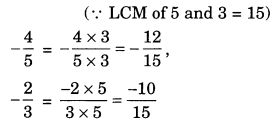Since there is only one integer i.e. -11 between -12 and -10, we have to find equivalent rational numbers.(iv) $$\frac{1}{2} \text { and } \frac{2}{3}$$
Converting each of the rational numbers in their equivalent rational numbers, we have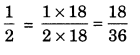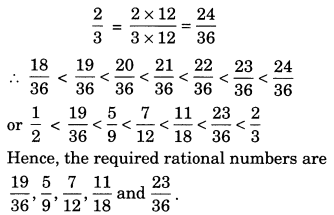Ex 9.1 Class 7 Maths Question 2.
Write four more rational numbers in each of the following patterns: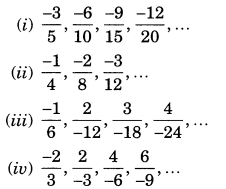Solution: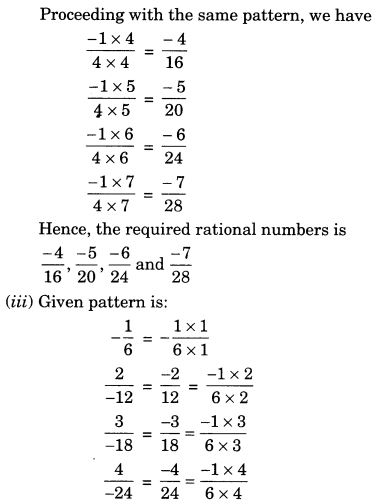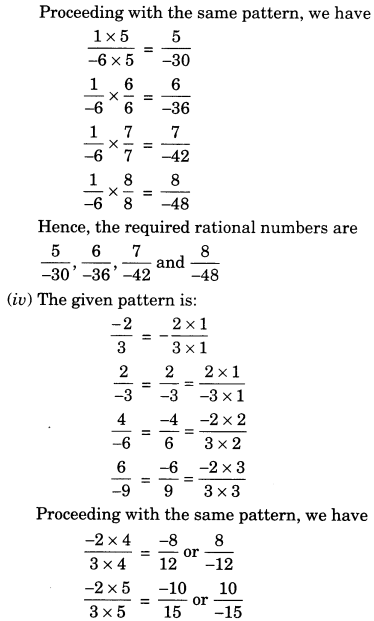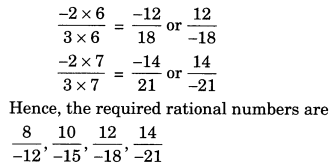Ex 9.1 Class 7 Maths Question 3.
Give four rational numbers equivalent to: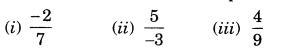Solution: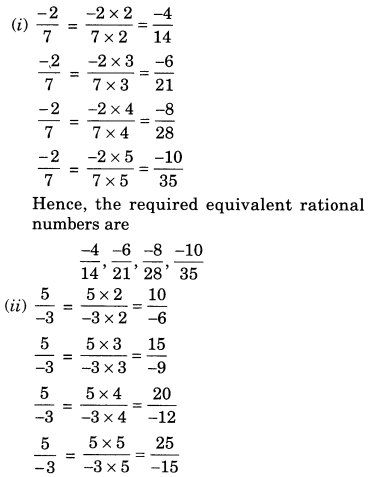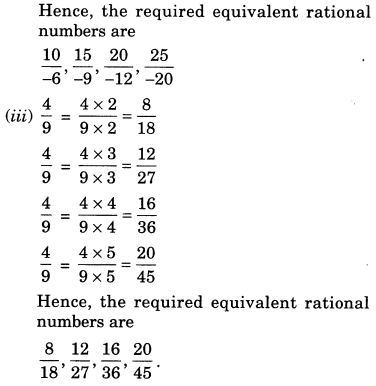Ex 9.1 Class 7 Maths Question 4.
Draw a number line and represent the following rational numbers on it:Solution:Ex 9.1 Class 7 Maths Question 5.
The points P, Q, R, S, T, U, A and B on the number line are such that, TR = RS = SU and AP = PQ = QB. Name the rational numbers represented by P, Q, R and S.Solution:
Rational numbers represented by P, Q, R and S.
$$\frac{7}{3}, \frac{8}{3}, \frac{-4}{3} \text { and } \frac{-5}{3}$$ respectlvely.

Ex 9.1 Class 7 Maths Question 6.
Which of the following pairs represent the same rational number?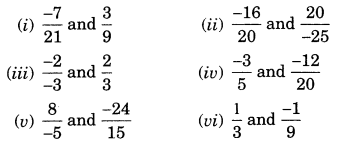Solution: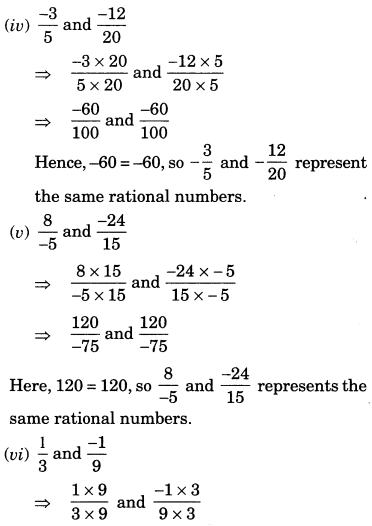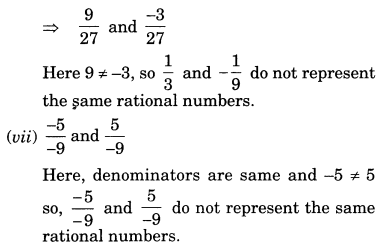Ex 9.1 Class 7 Maths Question 7.
Rewrite the following rational numbers in the simplest form:Solution: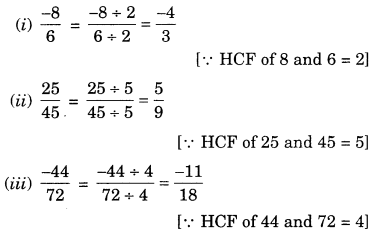Ex 9.1 Class 7 Maths Question 8.
Fill in the boxes with the correct symbol out of >, < and =.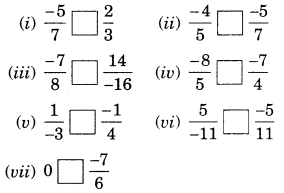Solution: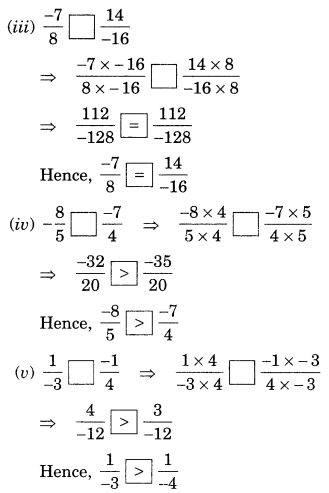Ex 9.1 Class 7 Maths Question 9.

Which is greater in each of the following: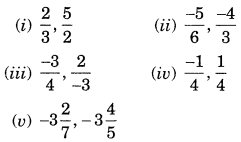Solution: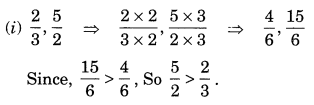Ex 9.1 Class 7 Maths Question 10.
Write the following rational numbers in ascending order:Solution: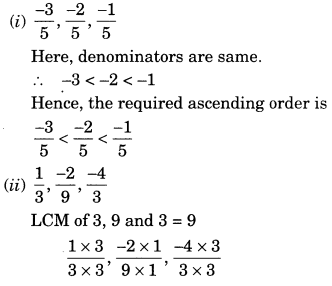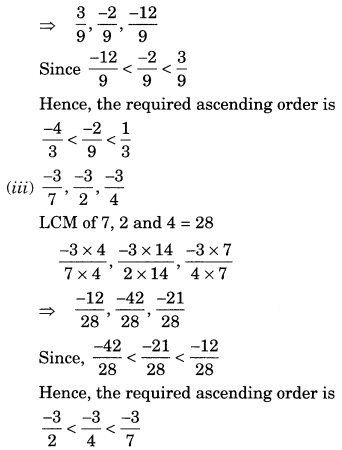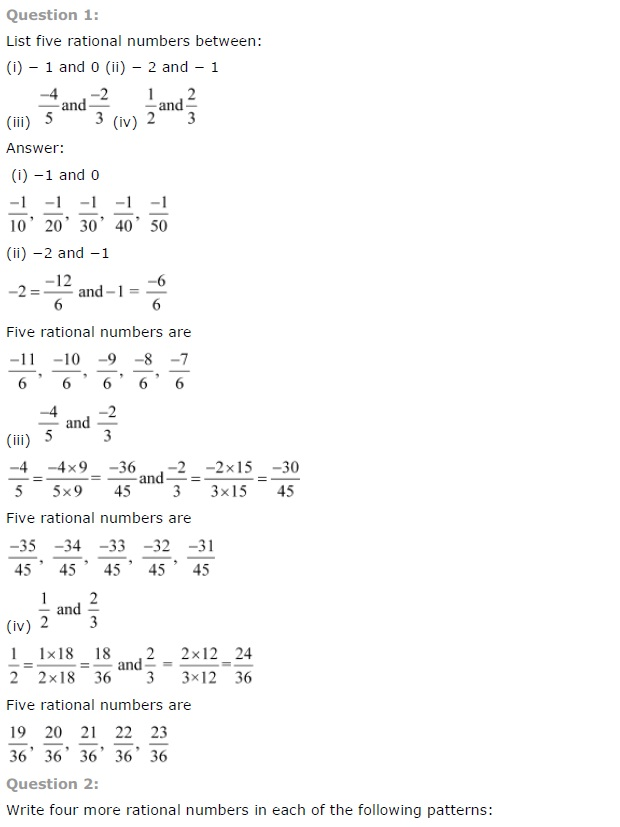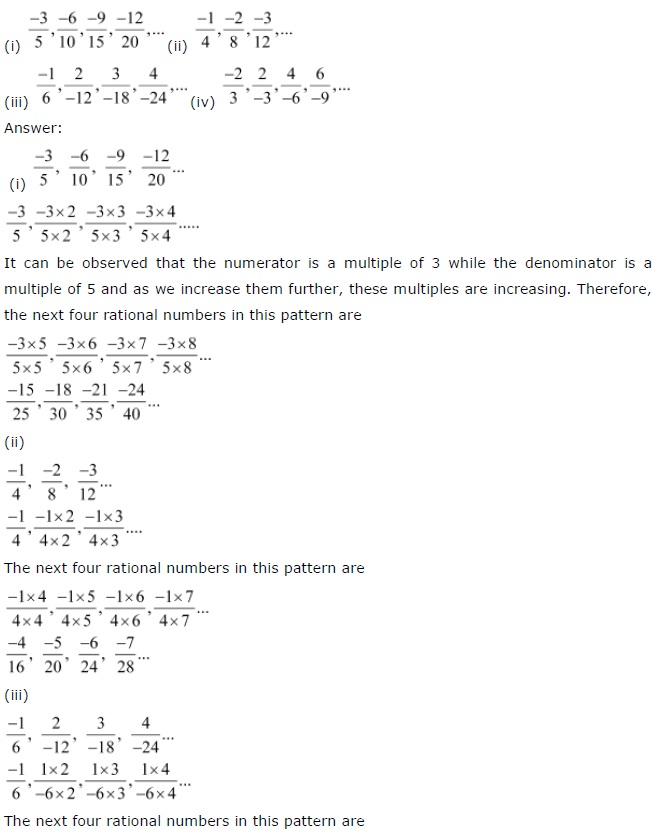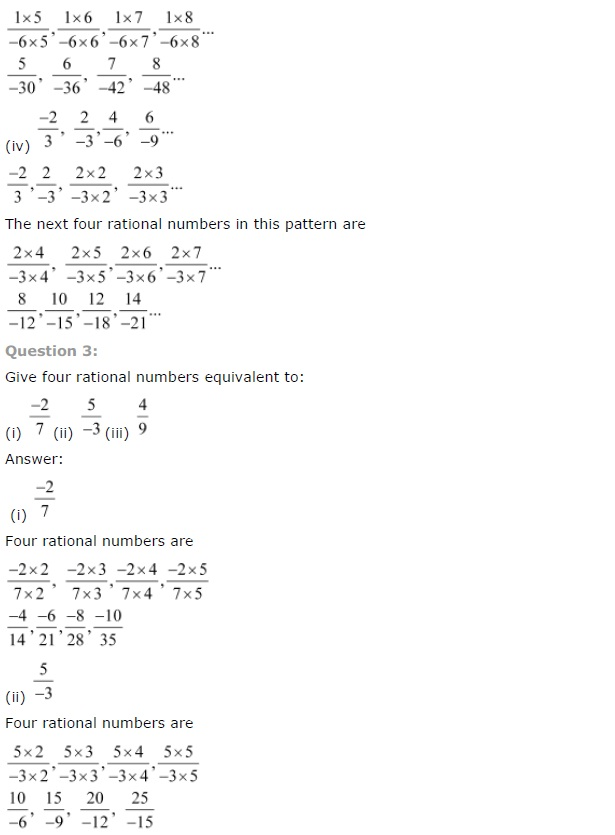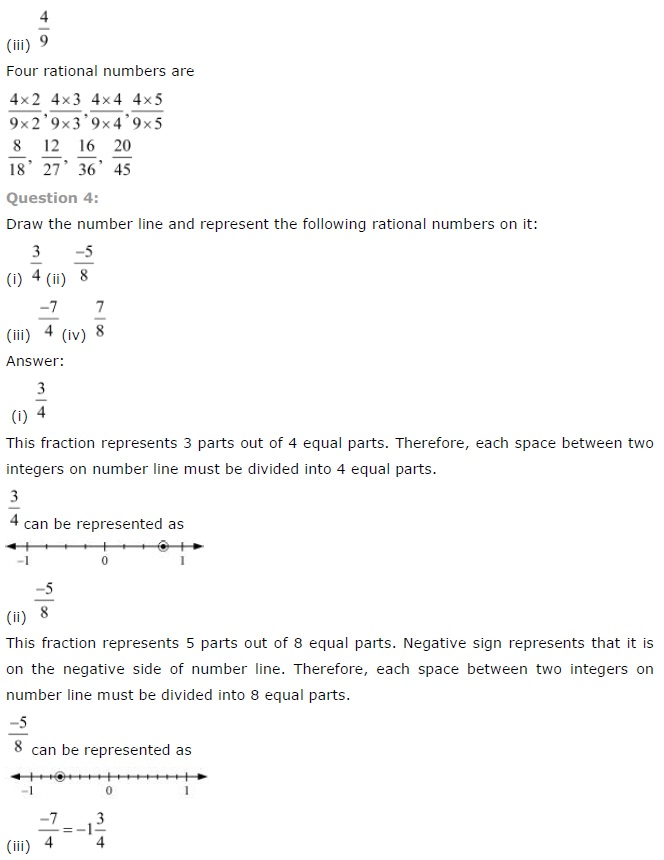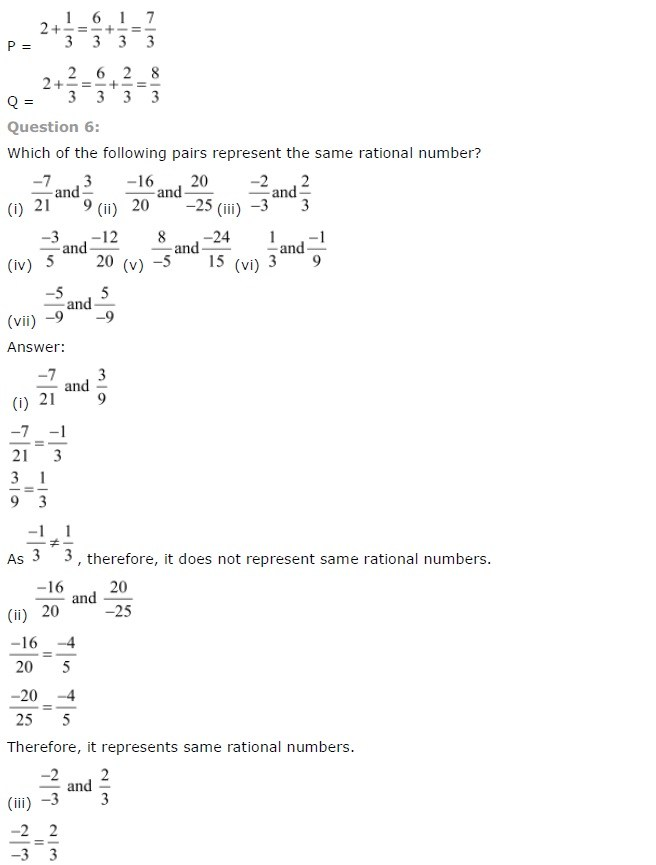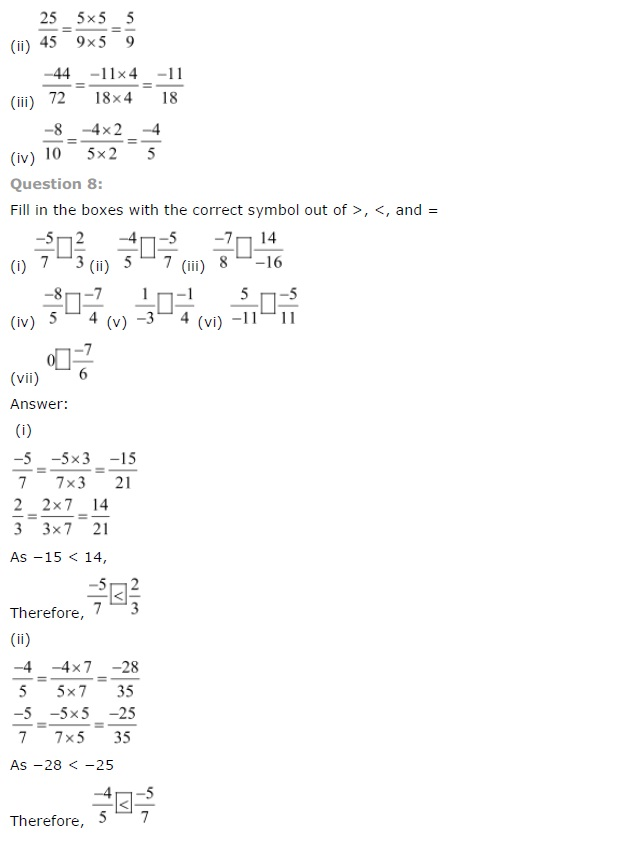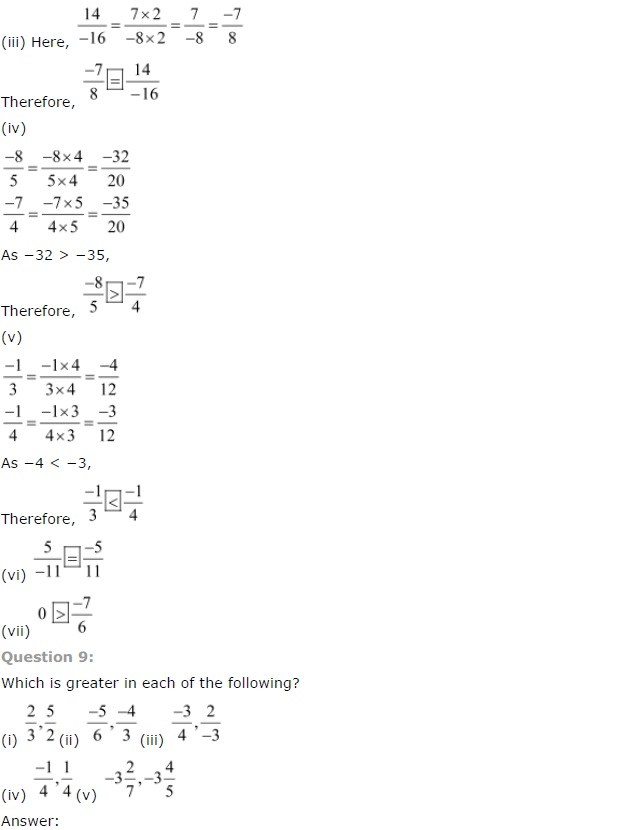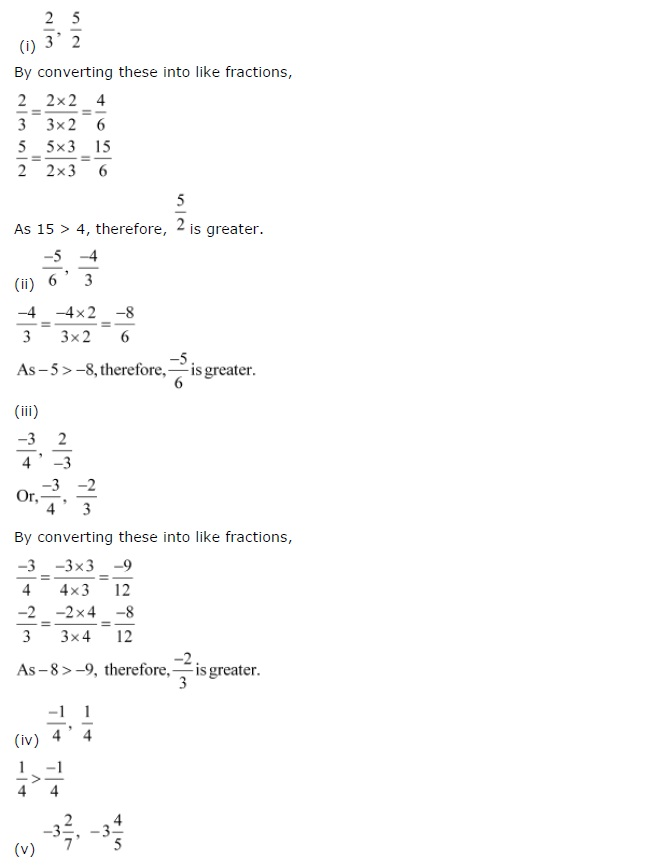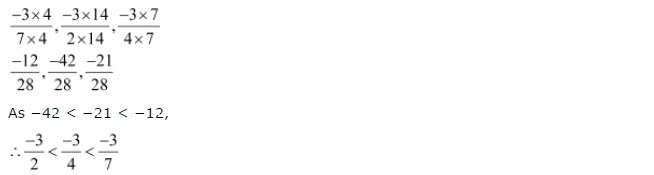Class 7 Maths Chapter 9 Exercise 9.2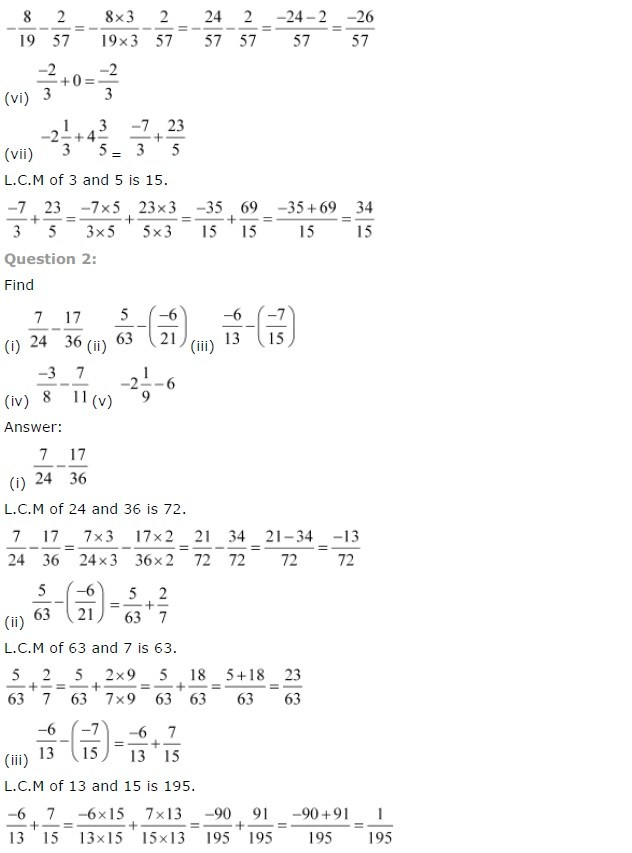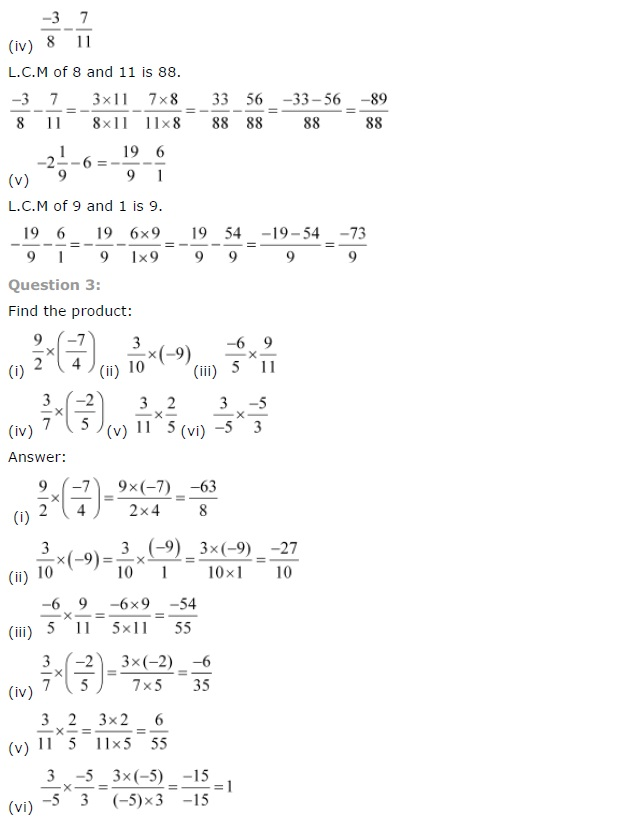+